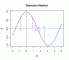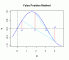I have forgotten

•http://facebook.com/
•https://www.google.com/accounts/o8/id
•https://me.yahoo.com

# Rootfinding

#### Bernoulli

Calculates the zeros of a polynomial using Bernoulli's algorithm.
 std::vector bernoulli (const std::vector &polynomial)

#### Aitken

Calculates the zeros of a function using Aitken acceleration.
 double aitken (double (*f)(double), double x0 = 0, double eps = 1E-10, int maxit = 1000, double c = -1)

#### Bisection

Calculates the zeros of a function using the bisection method.
 double bisection (double (*f)(double), double x0 = -1E+7, double x1 = 1E+7, double eps = 1E-10)#### Brent

Calculates the zeros of a function using Brent's method.
 double brent (double (*f)(double), double x1 = -1E+7, double x2 = 1E+7, double eps = 1E-10, int maxit = 1000)

#### Falseposition

Calculates the zeros of a function using the Regula-Falsi method.
 double falseposition (double (*f)(double), double x0 = -1E+7, double x1 = 1E+7, double eps = 1E-10, double maxit = 1000)#### Muller

Calculates the zeros of a function using Muller's method.
 double muller (double (*f)(double), double x0 = 0, double d = 3, double eps = 1E-10, int maxit = 1000)

#### Newton

Calculates the zeros of a function using Newton's method.
 double newton (double (*f)(double), double (*df)(double), double x = 0, double eps = 1E-10, int maxit = 1000)#### Secant

Calculates the zeros of a function using the secant method.
 double secant (double (*f)(double), double x0 = -1E+7, double x1 = 1E+7, double eps = 1E-10, int maxit = 1000)